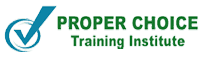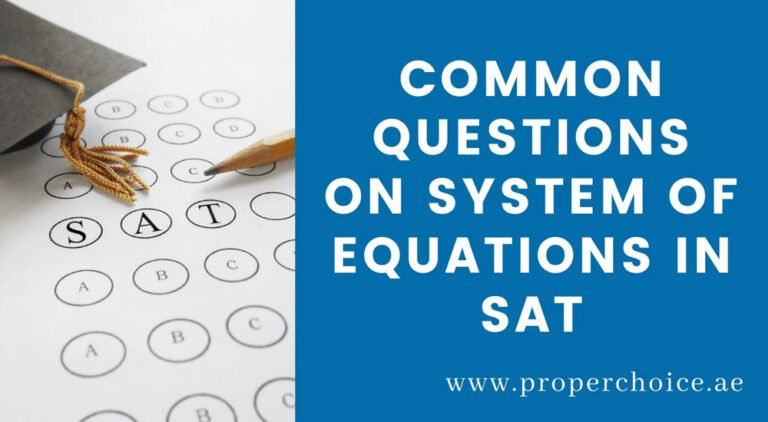# Most Common Questions on System of equation in SAT Test### Most Common Questions on System of equation in SAT Test

The system of equation is an important topic as per SAT Test concern, so it is very important to know the types of questions students can expect in the exam.

The student may be asked to determine the value of a constant or the coefficient of an equation in which the system has no solution, one solution, or infinitely many solutions.

Here are the three most important ways to analyze the given system of equation in two variables.

READ ALSO: Best Way to Prepare For SAT 2020

### No Solution Equations

Let a1x + b1y = c1and a2x + b2y = c2are the system of equations in whicha1, a2, b1, b2, c1and c2are constants, the equation has no solution if and only if

` a1= a2  b1= b2  c1 ≠c2 `

Let’s look at the following equation:

` 5x + 2y = 4   5x + 2y = 9 `

The coefficient of variable x is the same and the coefficient of variable y is also the same in both equations but the constant terms are different, so the system of equation has no solution.

Let’s analyze to see how it works!

To solve the given system of equation, subtract equation (1)-(2) which gives 0 = -5. However, something odd happens that can’t be right! We know that zero doesn’t equal to negative five. It is a false statement to say 0 = -5, so we know that there can be no solution.

Note: System of equation which does not have solutions are parallel lines, parallel lines never meet, so no solution.

Example: If the system of equation has no solution what is the value of k?

` kx + 8y = 10  x + 2y = 9  `

As mention, the system of equation has no solutions meaning the coefficients of variable x must be the same and also the coefficient of variable y must be the same in both equations. But the given equation doesn’t have the same coefficient of y, let’s make it by multiplying the second equation by 4.

` kx + 8y = 10  4x + 8y = 36   Then the equation becomes   (2x + 2y = 9) x 4  8x + 8y = 36  `

If coefficients of y in both equations are the same than a coefficient of x must also be same, so we can say that the value of k=4

### One Solution Equations

Let a1x + b1y = c1 and a2x + b2y = c2are the system of equations whicha1, a2, b1, b2, c1and c2are constants, the equation has one solution if and only if

` a1≠ a2  b1≠ b2  c1 ≠c2 `

There are two different methods to solve the system of equations in two variables, one is the Elimination method and another is the Substitution method. You can use any of these two methods to solve for x and y.

Let’s look at the following example and solve for variable x and y by using elimination method

` 5x + 4y = 8    2x + 2y = 3 `

To eliminate term y, let’s multiply second equation by 2 and change the sign of resulting equation (you can also multiply second equation by negative 2)

` (2x + 2y = 3) x2  4x + 4y = 6 Now subtract equation (1) - (3)   5x + 4y = 8  4x + 4y = 6  (-) (-) (-)  x = 2  `

Substitute the value of x in either equation (1) or equation (2) to get the value of y

Let’s substitute in equation (2)

` 2(2) + 2y= 3  2y= 3 - 4  2y= -1  y= -  1/2`

Therefore the system of equation has (2, -1/2 )

Note: Intersecting lines has one solution

MAYBE YOU LIKE: SAT Exam FAQs [ Expert Guidance ]

Share

Related Posts